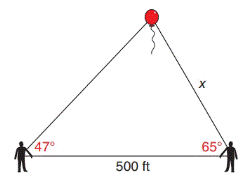Chapter 11.4, Problem 30E### Elementary Geometry for College St...

6th Edition
Daniel C. Alexander + 1 other
ISBN: 9781285195698

#### Solutions

Chapter
Section### Elementary Geometry for College St...

6th Edition
Daniel C. Alexander + 1 other
ISBN: 9781285195698
Textbook Problem
1 views

# In Exercises 29 to 34, use the Law of Sines or the Law of Cosines to solve each problem. Angle measures should be found to the nearest degree and areas and distances to the nearest tenth of a unit.Phil and Matt observe a balloon. They are 500  ft apart, and their angles of observation are 47 ∘ and 65 ∘ , as shown. Find the distance x from Matt to the balloon.To determine

To find:

The distance x from matt to the balloon.

Explanation

Formula:

The Law of sines,

sinαa=sinβb=sinγc

Where α, β, and γ is the angle of the triangle, and a, b, and c is the sides of the triangle.

Calculation:

Given,

Phil and Matt observe a balloon.

They are 500 ft apart, and their angles of observation are 47 and 65 are shown below.

Let A and B were Phil and Matt’s position respectively and C is the balloon’s position with angle α.

Then, AB¯=500 ft , BC¯=x , B=65 , and A=47

a=500 ft , b=x , β=65 , and γ=47

Find x:

For any triangle,

α+β+γ=180

Here,  β=65  and  γ=47

α+47+65=180

α+112=180

### Still sussing out bartleby?

Check out a sample textbook solution.

See a sample solution

#### The Solution to Your Study Problems

Bartleby provides explanations to thousands of textbook problems written by our experts, many with advanced degrees!

Get Started

#### Find more solutions based on key concepts